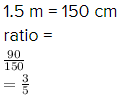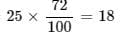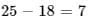Test: Comparing Quantities

# Test: Comparing Quantities

Test Description

## 15 Questions MCQ Test Mathematics (Maths) Class 7 | Test: Comparing Quantities

Test: Comparing Quantities for Class 7 2022 is part of Mathematics (Maths) Class 7 preparation. The Test: Comparing Quantities questions and answers have been prepared according to the Class 7 exam syllabus.The Test: Comparing Quantities MCQs are made for Class 7 2022 Exam. Find important definitions, questions, notes, meanings, examples, exercises, MCQs and online tests for Test: Comparing Quantities below.
Solutions of Test: Comparing Quantities questions in English are available as part of our Mathematics (Maths) Class 7 for Class 7 & Test: Comparing Quantities solutions in Hindi for Mathematics (Maths) Class 7 course. Download more important topics, notes, lectures and mock test series for Class 7 Exam by signing up for free. Attempt Test: Comparing Quantities | 15 questions in 10 minutes | Mock test for Class 7 preparation | Free important questions MCQ to study Mathematics (Maths) Class 7 for Class 7 Exam | Download free PDF with solutions
 1 Crore+ students have signed up on EduRev. Have you?
Test: Comparing Quantities - Question 1

### Find the ratio of 3 km to 300 m.

Detailed Solution for Test: Comparing Quantities - Question 1

First convert both the distance to the same unit
So 3 km = 3×1000m
= 3000 m
Thus the required ratio, 3 km : 300 m is 3000 : 300 = 10 : 1

Test: Comparing Quantities - Question 2

### A map is given with a scale of 2 cm = 1000 km. What is the actual distance between the two places in kms, if the distance in the map is 2.5 cm?

Detailed Solution for Test: Comparing Quantities - Question 2

Since 2 cm = 1000km
1 cm = 500km
2.5cm = 500*2.5
=1250km

So option C is the correct answer

Test: Comparing Quantities - Question 3

### 6 bowls cost Rs 90. What would be the cost of 10 such bowls?

Detailed Solution for Test: Comparing Quantities - Question 3

6 bowls cost Rs.90
so 1bowl cost 90/6=Rs.15
cost of 10 bowls=10×15=Rs.150

So option A is the correct answer.

Test: Comparing Quantities - Question 4

The car that I own can go 150 km with 25 litres of petrol. How far can it go with 30 litres of petrol?

Detailed Solution for Test: Comparing Quantities - Question 4

Distance covered with 25 litres of petrol = 150 Km

Distance covered with 30 Litres of petrol= 150/25 × 30 = 180 Km (Using Unitary Method)

Test: Comparing Quantities - Question 5

The ratio of 90 cm to 1.5 m is

Detailed Solution for Test: Comparing Quantities - Question 5So option C is the correct answer.

Test: Comparing Quantities - Question 6

A picnic is being planned in a school for Class VII. Girls are 60% of the total number of students and are 18 in number. The ratio of the number of girls to the number of boys in the class is

Detailed Solution for Test: Comparing Quantities - Question 6

Let total students be x
60/100* x = 18
x = 30
boys = 30−18 = 12
ratio of girls/ ratio of boys = 18 /12 = 3/2

So option C is the correct answer.

Test: Comparing Quantities - Question 7

Find the ratio of Speed of a cycle 15 km per hour to the speed of scooter 30 km per hour.

Detailed Solution for Test: Comparing Quantities - Question 7

Speed of cycle = 15km/hr
Speed of scooter = 30km/hr
Ratio = cycle/scooter
= 15/30
= 1/2

So option C is the correct answer.

Test: Comparing Quantities - Question 8

Find the ratio of 5 m to 10 km.

Detailed Solution for Test: Comparing Quantities - Question 8

Ratio of 5m to 10km is

10km = 10000m

5 : 10000

1 : 2000

So option C is the correct answer.

Test: Comparing Quantities - Question 9

Find the ratio of 50 paise to Rs 5.

Detailed Solution for Test: Comparing Quantities - Question 9

Explanation

we have to find 50 Paisa to 5 rupees

we know that 1 rupee = 100 Paisa

therefore 5 rupees = 100 × 5 = 500 Paisa

hence ratio between them :-

=> 50 : 500

=> 1 : 10

So option B is the correct answer.

Test: Comparing Quantities - Question 10

72% of 25 students are good in Mathematics. How many are not good in Mathematics?

Detailed Solution for Test: Comparing Quantities - Question 10

Number of students good in Math =So, number of students not good in Math =Test: Comparing Quantities - Question 11

Convert 1/8 to percent

Detailed Solution for Test: Comparing Quantities - Question 11

⅛*100=½*25=12.5%. For fraction to percentage , we multiply the fraction by 100

Test: Comparing Quantities - Question 12

What is  75% of 1 kg

Detailed Solution for Test: Comparing Quantities - Question 12

75% of 1kg

= 75/100 x 1000

= 750

So option C is the correct answer.

Test: Comparing Quantities - Question 13

What will be  5% to fractions in simplest form?

Detailed Solution for Test: Comparing Quantities - Question 13

5% can be written as  5 / 100 = 1/20

So option D is the correct answer.

Test: Comparing Quantities - Question 14

Find the ratio of 15 kg to 210 g

Detailed Solution for Test: Comparing Quantities - Question 14

We know that 1kg = 1000 g

Given, 15kg  = 15000 g

15kg to 210g = 15000 : 210

= 15000/210

= 500 : 7.

Test: Comparing Quantities - Question 15

Convert 20% to fractions in simplest form will be

Detailed Solution for Test: Comparing Quantities - Question 15

We know 20% can be written as :

20 / 100 = 1 / 5

So option D is the correct answer.

## Mathematics (Maths) Class 7

168 videos|252 docs|45 tests
 Use Code STAYHOME200 and get INR 200 additional OFF Use Coupon Code
Information about Test: Comparing Quantities Page
In this test you can find the Exam questions for Test: Comparing Quantities solved & explained in the simplest way possible. Besides giving Questions and answers for Test: Comparing Quantities, EduRev gives you an ample number of Online tests for practice

## Mathematics (Maths) Class 7

168 videos|252 docs|45 tests